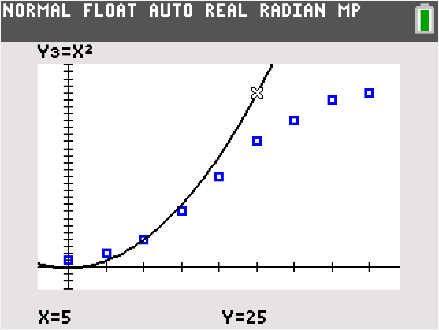••• ##### Device
•TI-84 Plus C Silver Edition
•TI-84 Plus CE
• ##### Software

TI-SmartView™ CE

# Mathematical Modeling: Modeling Virus Spread

by Texas Instruments#### Objectives

Students will be able to:

• Model a contextual situation mathematically and use the model to answer a question
• Use data from a simulation to create a scatter plot, and describe how a variable changes over time
• Determine and interpret a function modeling a quantitative variable over time